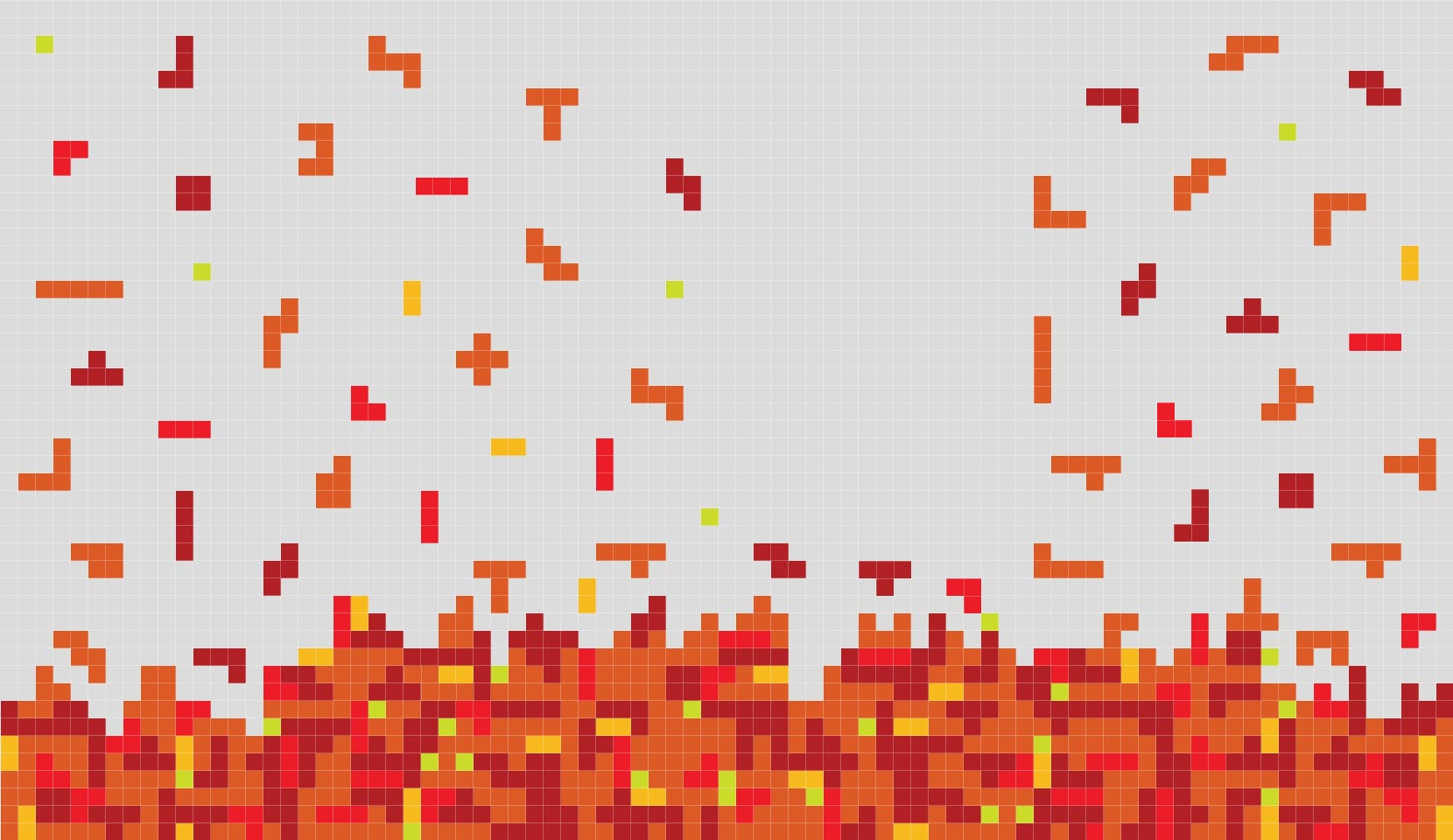Abstract

Non classical logics provide adequate formalisms for reasoning, e.g., about time, knowledge, dynamic data structures, vague information, resources and algebraic varieties. As such, these logics have been increasingly applied in various disciplines including artificial intelligence, mathematics, and in computer science, where most of these logics originate.

Non classical logics are usually introduced or described in a declarative way in the proof framework due to Hilbert and Frege. However, the resulting systems are extremely cumbersome when it comes to finding or analyzing proofs. Moreover, a Hilbert-Frege system does not help answer useful questions about the formalized logic and the corresponding algebraic structure, such as 'Is the logic decidable?' or 'Does the algebra satisfy amalgamation or a certain order theoretic completion?'. These tasks call for algorithmic presentations of the logics especially in the form of analytic calculi. Analyticity is crucial here since proofs in such calculi proceed by a stepwise decomposition of the objects to be proved. The construction of analytic calculi for non classical logics has long been tailored to each specific logic and the solutions to fundamental questions about these logics and related algebras (decidability, complexity, ...) have largely been investigated on an individual basis.

Our aim is to develop theoretical and engineering tools for automatically extracting analytic calculi for non classical logics from Hilbert-style systems. The resulting tools will be utilized to systematically investigate non classical logics. Various results, currently proved in a logic-specific fashion, will be established in a uniform way for large classes of logics. Our focus will not be on particular calculi, logics or algebras, but on general and uniform results whose instantiations are expected (i) to help logic users in the spirit of 'logic engineering', and (ii) to solve open questions on specific logics, calculi or algebras.